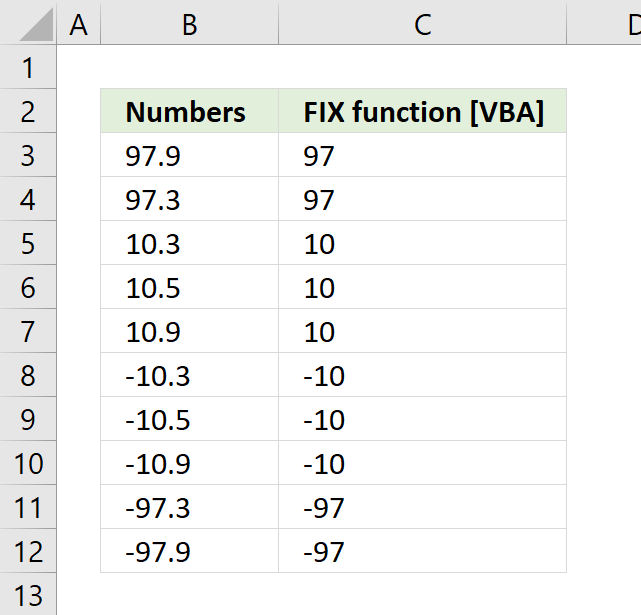Author: Oscar Cronquist Article last updated on August 29, 2018The FIX function removes the decimals from the argument.

Fix(number)

### Arguments

 number Required. Is a Double or any valid numeric expression. If number is 0 (zero) then 0 (zero) is returned.

### Comments

I used the following macro to show how the Fix function behaves with different arguments.

```Sub Macro1()
For Each cell In Range("B3:B12")
cell.Offset(, 1) = Fix(cell.Value)
Next cell
End Sub
```

### Formula equation

Fix(number) = Sgn(number) * Int(Abs(number))